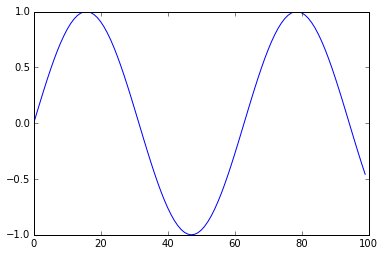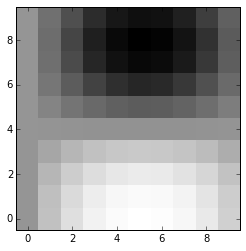# Introduction to Neural Networks (Psy 5038): Python¶

So far in this course we've tried to emphasize concepts usually with toy examples. We'll now spend a few classes going over tools that can be applied to state-of-the-art problems in cognitive neuroscience. Mathematica is excellent for learning concepts, and for many high-end applications. However, it is not so good for specialized work in the field. Matlab and increasingly Python have large user communities who are building tools that we can build on. That's the goal. But first an introduction to IPython as an environment for scientific programming.

## Starting IPython in the middle¶

In order to run this notebook on your computer, you will need to have Python and IPython installed. Find the directory where you've downloaded this notebook, and then from a terminal command line go the directory (or parent directory) and type: ipython notebook. This will bring up a browswer window from where you should see this notebook listed in your browser window. You can load it from there.

###### Installation¶

The hardest, or at least the most frustrating, aspect of Python can be installation. There are a number of package managers. We recommend Anaconda.

In addition to Python and IPython, you will need numpy, matplotlib and the scipy library. All of these are part of the scipy stack for general purpose scientific programming. Later, we'll also need pymc for Bayesian computations using MCMC sampling, the machine learning module scikit-learn, and scikit-image, which are built on the scipy stack.

These installation notes from a 2013 course at Harvard may be helpful.

### Style¶

Let's first cover some style. We'll get to substance--i.e. python code--soon enough. Right off the bat, you can create a Raw NBConvert, various headings, or a Markdown cell. Try the latter and type in LaTeX commands. E.g. try putting the next line between double dollar signs:

p(y_i|x)= \frac{e^{y_i(w.x+b)}}{{1+e^{(w.x+b)}}}

After getting acquainted with the menu items and buttons of the IPython notebook interface, take a look at these notes on: IPython's Rich Display System. Try copying in cell content in this notebook to try out displaying different kinds of content.

### Making and plotting lists¶

In :
import numpy as np


To get started, let's look at some simple python coding examples. We need to load numpy to handle vectors and matrices. To make lists in Mathematica we typically used Table[], e.g.

Table[Sin[x], {x, 0, 1, .1}];



In python, we'll use "list comprehensions". Create a code cell and try these:

[sin(x) for x in arange(0,1,.1)]

[sin(x) for x in linspace(0,1,10)]



But wait! Python needs to know where these functions came from. arange(), linspace(), and sin() are all numpy functions. We imported numpy functions with the shorthand "np", so you will need to write:

np.sin(x), and np. arange(0,1,.1).



For more on creating and manipulating numerical lists in NumPy see scipy page

In :
sl=[np.sin(x) for x in np.arange(0,10,.1)]


Let's plot these values. To do this, we'll need to import matplotlib, and in particular the pyplot module, or plt for short. If you want a more matlab like plotting environment, you can use pylab:

from pylab import *



For more information on plotting, see scipy notes, and Lecture 4 in the scientific python notebook series.

In :
import matplotlib.pyplot as plt

In :
plt.plot(sl)
plt.show()With the magic function the next cell, your plot should appear in a separate window. Close the figure window and now excecute the next cell below, and then run the above plot cell again.

In :
%matplotlib inline


We made 2D lists in Mathematica using the Table[] function like this:

Table[Sin[x] Cos[y], {x, 0, 1, .1}, {y, 0, 1, .1}];



Now try using the list comprehension syntax for python:

[[sin(x)*cos(y) for x in arange(0,1,.1)] for y in arange(0,1,.1)] or

[[sin(x)*cos(y) for x in linspace(0,1,10)] for y in linspace(0,1,10)]



Again remember to specify the numpy class using np.

In :
#If you remove the semicolon and it is the last line, the next line will print out the values
[[np.sin(x)*np.cos(y) for x in np.arange(0,1,.1)] for y in np.arange(0,1,.1)];

In :
#but it won't if you assign a variable name to the array

sl2=[[np.sin(x)*np.cos(y) for x in np.arange(0,1,.1)] for y in np.arange(0,1,.1)];

###### Getting parts of vectors and arrays¶
In :
#make a vector with 5 rows and 6 columns
sl3=np.array([[x+y*6 for x in np.arange(0,6,1)] for y in np.arange(0,5,1)])
#what happens if you don't use np.array() in the above line?

print np.array(sl3),'\n\n(#rows, #columns)=',np.shape(sl3),"# elements:",np.size(sl3)

[[ 0  1  2  3  4  5]
[ 6  7  8  9 10 11]
[12 13 14 15 16 17]
[18 19 20 21 22 23]
[24 25 26 27 28 29]]

(#rows, #columns): (5, 6) # elements: 30

In :
#note that unlike Mathematica and Matlab (but like C), indexing starts with 0
#first row
sl3

In :
#first column
sl3[:,0]

Out:
array([ 0,  6, 12, 18, 24])
In [ ]:
#Try accessing the element in the 3rd row, 4th column. It isn't sl3[3,4]|

#Get the first 2 elements of sl. It isn't sl[0:1].

In :
#Now get the submatrix 3rd and 4th row, 4th through 6th columns
sl3[2:4,3:6]

Out:
array([[15, 16, 17],
[21, 22, 23]])
###### Defining functions¶
In :
def f(x,y):
return([[np.sin(3*x)*np.cos(4*y) for x in np.arange(0,1,.1)] for y in np.arange(0,1,.1)])

In :
#Visualize f(x,y) using imshow()

import matplotlib.cm as cm

n = 10
x = np.linspace(-3,3,4*n)
y = np.linspace(-3,3,3*n)
X, Y = np.meshgrid(x,y)
plt.imshow(f(X,Y),cmap = cm.Greys_r,origin='lower',interpolation='nearest');###### IPython Examples¶

For a directory of IPython notebook examples. E.g. here's a demo of receptive field models

###### Python neural network resources¶

For python code, look at neurolab. This has several topics that should look familiar. However, there is some material that we haven't covered, for example see Elmans network for learning temporal sequences.

For python code to simulate spiking neurons, see http://briansimulator.org.

In [ ]:



Ok, now let's look at a neural network. We will download a python file that simulates the two neuron Hopfield net TwoNeuroHopfield.py. And then convert it to an IPython notebook.

For the IPython version, look at this link

In [ ]: